# Quiz 5: Theory of Consumer Behavior

Marginal utility is the additional satisfaction derived from the consumption of one more unit of a good or a service. An indifference curve shows all the possible combinations of two goods or services which provide the same satisfaction level to the consumer. A higher indifference curve represents a higher level of utility. A budget line or a constraint shows all the combinations of goods or services purchased by the consumers, given the market price levels and their income level. It is written as follows: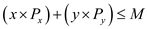Here, ' x ' = Good ' x ' ' y ' = Good ' y ' ' Px ' = Price of ' x ' ' P y ' = Price of ' y ' ' M ' = Total income The optimal consumption bundle is given by the tangency of the budget line and the indifference curve. This reflects the fact that the optimal bundle is where the consumer gets maximum utility from consumption of both goods given the budget constraint of the consumer. At the point of tangency, the slopes of the budget line and the indifference curve are equal. That is, the following condition holds true:Here, ' MU x ' = Marginal utility of good ' x ' ' MU y ' = Marginal utility of good ' y ' Suppose that the marginal utility of wine and cheese are 50 units and 40 units respectively. The price of wine is \$10 per bottle and that of cheese is \$4 per pound. (a) The optimal point of marginal utility (MU) is when the ratio of marginal utility and price (P) of wine ( x ) and cheese ( y ) are equal. This is shown below as follows:The marginal utility per-dollar spent on cheese yields more satisfaction to the consumer in comparison to the marginal utility per-dollar spent on wine. This means that the consumer can increase utility by increasing consumption. As the price ratio is not equal to or is greater than the ratio of marginal utilities, this means that the consumer is not making the utility-maximizing choice. (b) The consumer should buy more of cheese and less of wine because if one more dollar is spent of cheese and one less dollar spent on wine, then the total expenditure will be unchanged but the utility will increase by 5 units. This could go on until both the price and marginal utilities ratios are equal for both goods.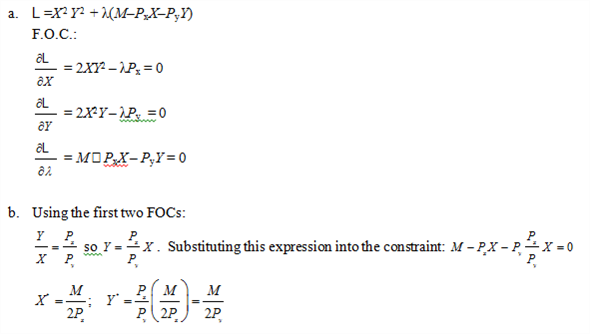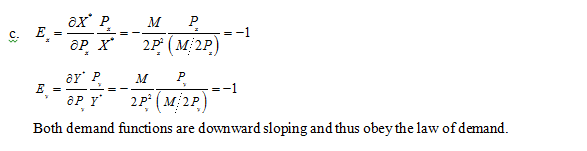Given 25 grams carbohydrate per cup for rice krispies 6 grams carbohydrate per cup for cheese 10grams carbohydrate per cup for popcorn The following table shows the marginal utility and price ratio of different products. The carbohydrate gram per cups is considered as price in order to determine the optimum combination.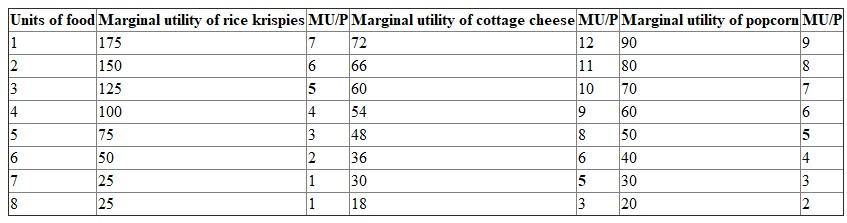a. Mr. B can consume 167 grams in following combination: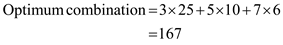Therefore, 7-cheese, 5-corn and 3-rice krispies should be consumed to maximize the 167 gram carbohydrate. b. The following equation shows the combination of food given bill can consume only 126 grams: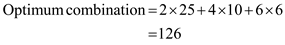Therefore, 6-cheese, 4-corn and 2-rice krispies should be consumed to get the 126 gram carbohydrate.